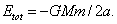# Gravity: the Essentials

Michael Fowler 2/15/07

Newton’s Law of Gravitation: be able to explain in what sense a cannonball’s flight is related to the Moon’s orbital motion.  Know that the magnitude of the gravitational force between two bodies of masses m1 and m2 a distance r apart F = Gm1m2/r2,How does this relate to g?

Kepler’s Laws: you should know all three: I  Planets move in ellipses with the sun at one focus; II  Planets sweep out equal areas in equal times;  III   T2/A3 is the same for all planets in the System.  Be able to derive the Third Law for circular orbits (but know the result for elliptic orbits), and to explain how the Second Law relates to angular momentum.

The Gravitational Field: be able to sketch the field lines for two unequal masses, in particular near the point where the field vanishes.  Be able to find the gravitational field from a ring of matter at points on the axis. Know how to show the field inside a uniform shell of matter is zero, then use tht fact to figure out how g changes on going down a deep mine.

Gravitational Potential Energy: be able to find the gravitational potential energy of a mass m outside a spherical mass M (much greater than m) by calculating the work needed to change r.  What happens in a deep mine?  Be familiar with the relation between kinetic energy and potential energy in a circular orbit, and be able to explain how that kinetic energy relates to the energy needed to escape.

Elliptic Orbits: First, be familiar with the ellipse: a, b, e.  For the orbits, you need to know the two basic facts:, andYou can remember these formulas by noting that they’re exactly the same as for circular orbits, with r replaced by a, and they’re pretty easy to derive for circular orbits.  Questions concerning the most efficient path for a spaceship between two planets are beat dealt with by taking an elliptical orbit, touching the two circles at its extremes, then use energy and angular momentum conservation as the ship goes from one extreme to the other.  You should be able to explain qualitatively how the slingshot works, by analyzing it in the frame of reference of Jupiter.

Binary Stars and Tides: be able to write down the equation of motion for a binary star system with circular orbits, and solve to find the orbital time, given the masses of the stars and their separation.   Be able to explain briefly why there are two tides a day, rather than one.

General Relativity: be able to state the Equivalence Principle, and use it to make a ballpark estimate of the deflection of light as it passes the Sun.